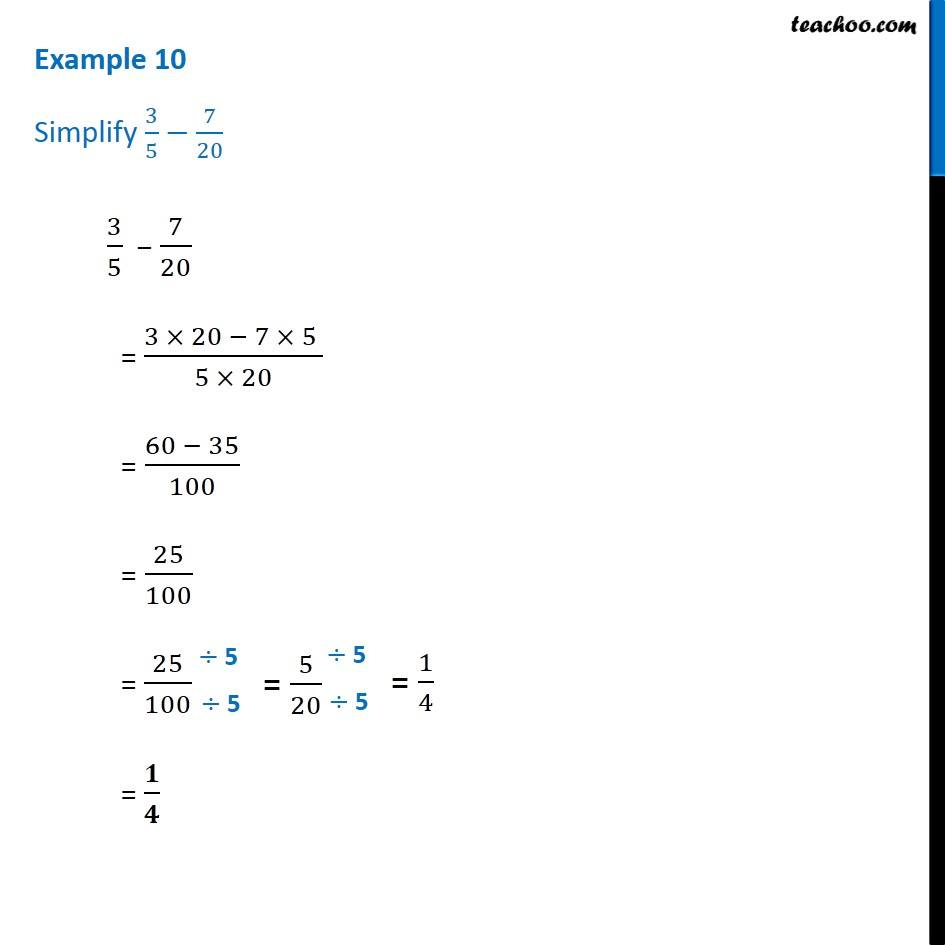Examples

Chapter 7 Class 6 Fractions
Serial order wiseLearn in your speed, with individual attention - Teachoo Maths 1-on-1 Class

### Transcript

Example 10 - Chapter 7 Class 6 Fractions - NCERT Book Simplify 3/5− 7/20 3/5 − 7/20 = (3×20 −7×5)/(5×20) =(60 −35)/100 = 25/100 = 1/4